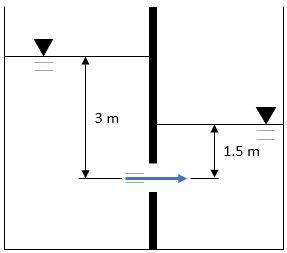## Submerged Orifice

In the shown figure, two water tanks are separated by a middle divider. If a square latch with 50 cm sides on the divider is opened such that water flows from the larger tank to the smaller tank, what is the flow rate through the latch in cubic meters per second? Assume there are no minor losses in the discharge, a common coefficient of discharge of 0.4, and steady-flow conditions.Hint
$$Q=CA\sqrt{2g(h_1-h_2)}$$$where $$Q$$ is the volumetric flow rate, $$C$$ is the coefficient of discharge, $$A$$ is the cross sectional area of flow, $$g$$ is the acceleration due to gravity, $$h_1$$ is the height of the fluid above the orifice for the taller portion, and $$h_2$$ is the height of the fluid above the orifice for the shorter portion. Hint 2 The cross sectional area for a square latch is: $$A=l \times l$$$
where $$l$$ is the side length.
Submerged orifice operating under steady flow-conditions:
$$Q=CA\sqrt{2g(h_1-h_2)}$$$where $$Q$$ is the volumetric flow rate, $$C$$ is the coefficient of discharge, $$A$$ is the cross sectional area of flow, $$g$$ is the acceleration due to gravity, $$h_1$$ is the height of the fluid above the orifice for the taller portion, and $$h_2$$ is the height of the fluid above the orifice for the shorter portion. $$Q=(0.4)(0.5m)^2\sqrt{2(9.8m/s^2)(3m-1.5m)}$$$
$$=(0.4)(0.25m^2)\sqrt{2(9.8m/s^2)(1.5m)}=(0.1m^2)\sqrt{29.4m^2/s^2}$$$$$=(0.1m^2)(5.422m/s)=0.542\:\frac{m^3}{s}$$$
$$0.542\:\frac{m^3}{s}$$\$